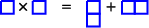$$\require{cancel}$$

# 7.7: Continuous Symmetries

•• Contributed by Niels Walet
• Professor (Physics) at University of Manchester

Rotational transformations on a wavefunction can be applied by performing the transformation

$\exp[i(\theta_x \hat J_x + \theta_y \hat J_y + \theta_z \hat J_z)]$

on a wavefunction. This is slightly simpler for a particle without spin, since we shall only have to consider the orbital angular momentum,

$\hat{\vec{L}} = \hat{\vec{p}} \times {\vec{r}} = {i\hbar} {\vec{r}} \times {\vec{\nabla}}.$

Notice that this is still very complicated, exponentials of operators are not easy to deal with. One of the lessons we learn from applying this operator to many different states, is that if a state has good angular momentum $$J$$, the rotation can transform it into another state of angular momentum $$J$$, but it will never change the angular momentum. This is most easily seen by labelling the states by $$J, M$$:

\begin{aligned} \left[{\hat J}_x^2+{\hat J}_y^2+{\hat J}_z^2\right] \phi_{JM} &= \hbar^2J(J+1) \phi_{JM}\\ {\hat J}_z^2 \phi_{JM} &= M \phi_{JM}\end{aligned}

The quantum number $$M$$ can take the values $$-J, -J+1,\ldots, J-1, J$$, so that we typically have $$2J+1$$ components for each $$J$$. The effect of the exponential transformation on a linear combination of states of identical $$J$$ is to perform a linear transformation between these components. I shall show in a minute that such transformation can be implemented by unitary matrices. The transformations that implement these transformations are said to correspond to an irreducible representation of the rotation group (often denoted by SO(3)).

A single Spin-1/2 particle

Let us look at the simplest example, for spin $$1/2$$. We have two states, one with spin up and one with spin down, $$\psi_\pm$$. If the initial state is $$\psi=\alpha_+ \psi_+ +\alpha_- \psi_-$$, the effect of a rotation can only be to turn this into $$\psi'=\alpha'_+ \psi_+ +\alpha'_- \psi_-$$. Since the transformation is linear (if I rotate the sum of two objects, I might as well rotate both of them) we find

$\left(\begin{array}{l} \alpha'_+ \\ \alpha'_- \end{array}\right) = \left(\begin{array}{ll} U_{++} & U_{-+}\\U_{+-}&U_{--} \end{array}\right) \left(\begin{array}{l} \alpha'_+ \\ \alpha'_- \end{array}\right)$

Since the transformation can not change the length of the vector, we must have $$\int |\psi'|^2 =1$$. Assuming $$\int |\psi_\pm|^2 =1$$, $$\int \psi_+^*\psi_- =0$$ we find

$U^\dagger U = 1$

with

$U^\dagger = \left(\begin{array}{ll} U^*_{++} & U^*_{+-}\\ U^*_{-+}&U^*_{--} \end{array}\right)$

the so-called hermitian conjugate.

We can write down matrices that in the space of $$S=1/2$$ states behave the same as the angular momentum operators. These are half the well known Pauli matrices

$\sigma_x = \left( \begin{array}{ll}0&1\\1 & 0 \end{array}\right),\;\; \sigma_y = \left( \begin{array}{ll}0&-i\\i & 0 \end{array}\right),\;\; \sigma_z = \left( \begin{array}{ll}1&0\\0 & -1 \end{array}\right).$

and thus we find that

$U({\vec{\theta}}) = \exp[i(\theta_x \sigma_x/2 + \theta_y \sigma_y/2 + \theta_z \sigma_z/2)].$

I don’t really want to discuss how to evaluate the exponent of a matrix, apart from one special case. Suppose we perform a $$2\pi$$ rotation around the $$z$$ axis, $${\vec{\theta}} = (0,0,2\pi)$$. We find

$U(0,0,2\pi) = \exp[i\pi \left( \begin{array}{ll}1&0\\0 & -1 \end{array}\right)].$

Since this matrix is diagonal, we just have to evaluate the exponents for each of the entries (this corresponds to using the Taylor series of the exponential),

\begin{aligned} U(0,0,2\pi) &= \left( \begin{array}{ll}\exp[i\pi]&0\\0 & \exp[-i\pi] \end{array}\right)\nonumber\\ &= \left( \begin{array}{ll}-1&0\\0 & -1\end{array}\right).\end{aligned}

To our surprise this does not take me back to where I started from. Let me make a small demonstration to show what this means....

Finally what happens if we combine states from two irreducible representations? Let me analyze this for two spin $$1/2$$ states,

\begin{aligned} \psi&=(\alpha^1_+ \psi^1_+ +\alpha^1_- \psi^1_-) (\alpha^2_+ \psi^2_+ +\alpha^2_- \psi^2_-) \nonumber\\ &= \alpha^1_+\alpha^2_+ \psi^1_+ \psi^2_++ \alpha^1_+\alpha^2_- \psi^1_+ \psi^2_- + \alpha^1_-\alpha^2_+ \psi^1_- \psi^2_+ \alpha^1_-\alpha^2_- \psi^1_- \psi^2_-.\end{aligned}

The first and the last product of $$\psi$$ states have an angular momentum component $$\pm1$$ in the $$z$$ direction, and must does at least have $$J=1$$. The middle two combinations with both have $$M=M_1+M_2=0$$ can be shown to be a combination of a $$J=1,M=0$$ and a $$J=0,M=0$$ state. Specifically,

$\frac{1}{\sqrt 2}\left[\psi^1_+ \psi^2_- - \psi^1_- \psi^2_+\right]$

transforms as a scalar, it goes over into itself. the way to see that is to use the fact that these states transform with the same $$U$$, and substitute these matrices. The result is proportional to where we started from. Notice that the triplet ($$S=1$$) is symmetric under interchange of the two particles, whereas the singlet ($$S=0$$) is antisymmetric. This relation between symmetry can be exhibited as in the diagrams Figure $$\PageIndex{1}$$ where the horizontal direction denotes symmetry, and the vertical direction denotes antisymmetry. This technique works for all unitary groups....Figure $$\PageIndex{1}$$: The Young tableau for the multiplication $$1/2\times1/2=0+1$$.

The coupling of angular momenta is normally performed through Clebsch-Gordan coefficients, as denoted by $\left\langle j_1 m_1 j_2 m_2| J M \right\rangle.$ We know that $$M=m_1+m_2$$. Further analysis shows that $$J$$ can take on all values $$|j_1-j_2|,|j_1-j_2|+1, |j_1-j_2|+2, j1+j2$$.